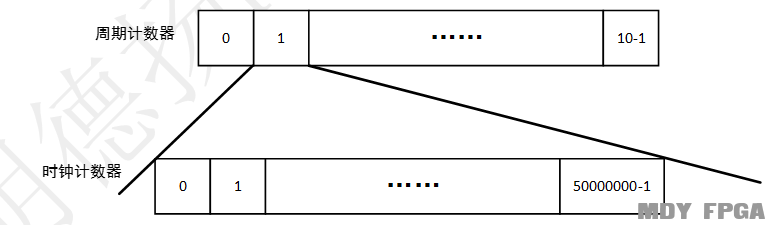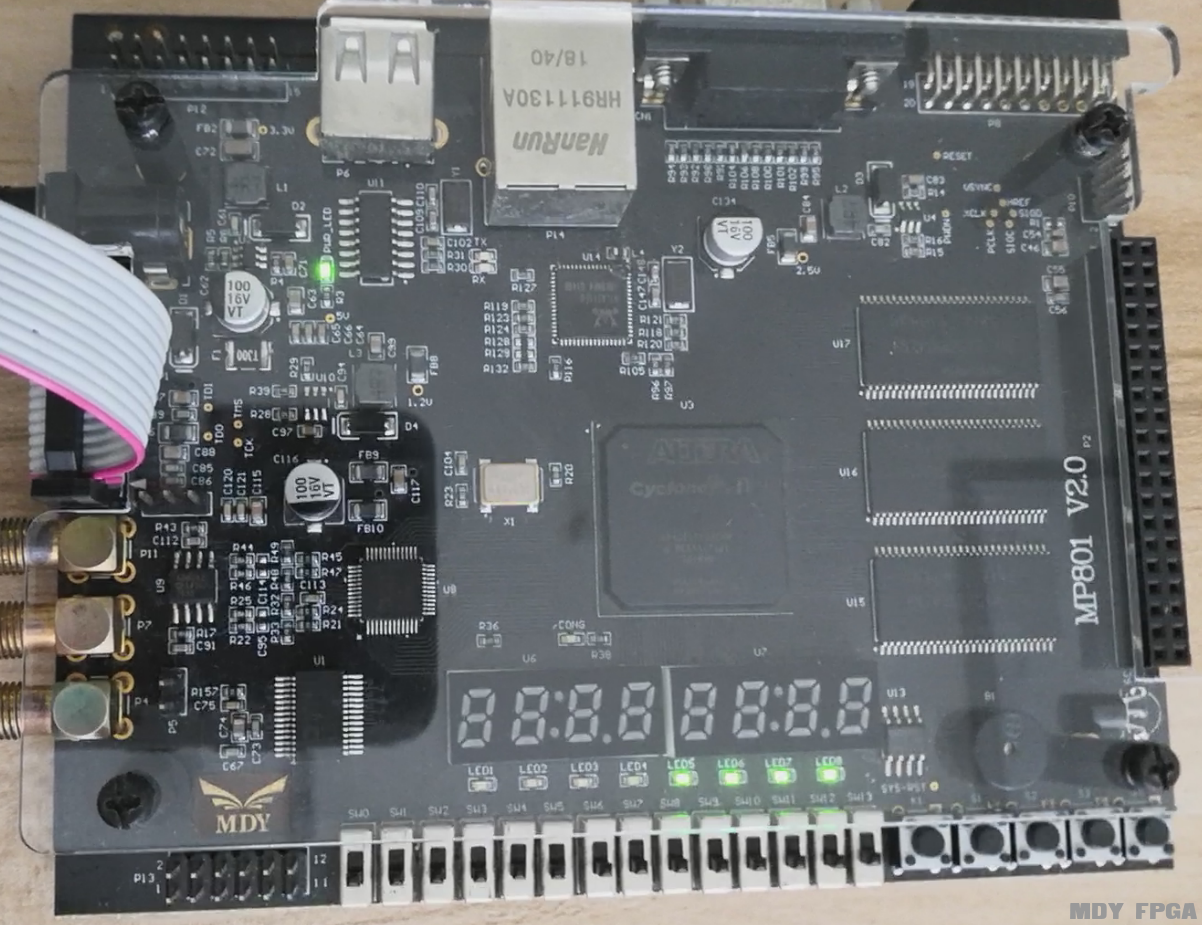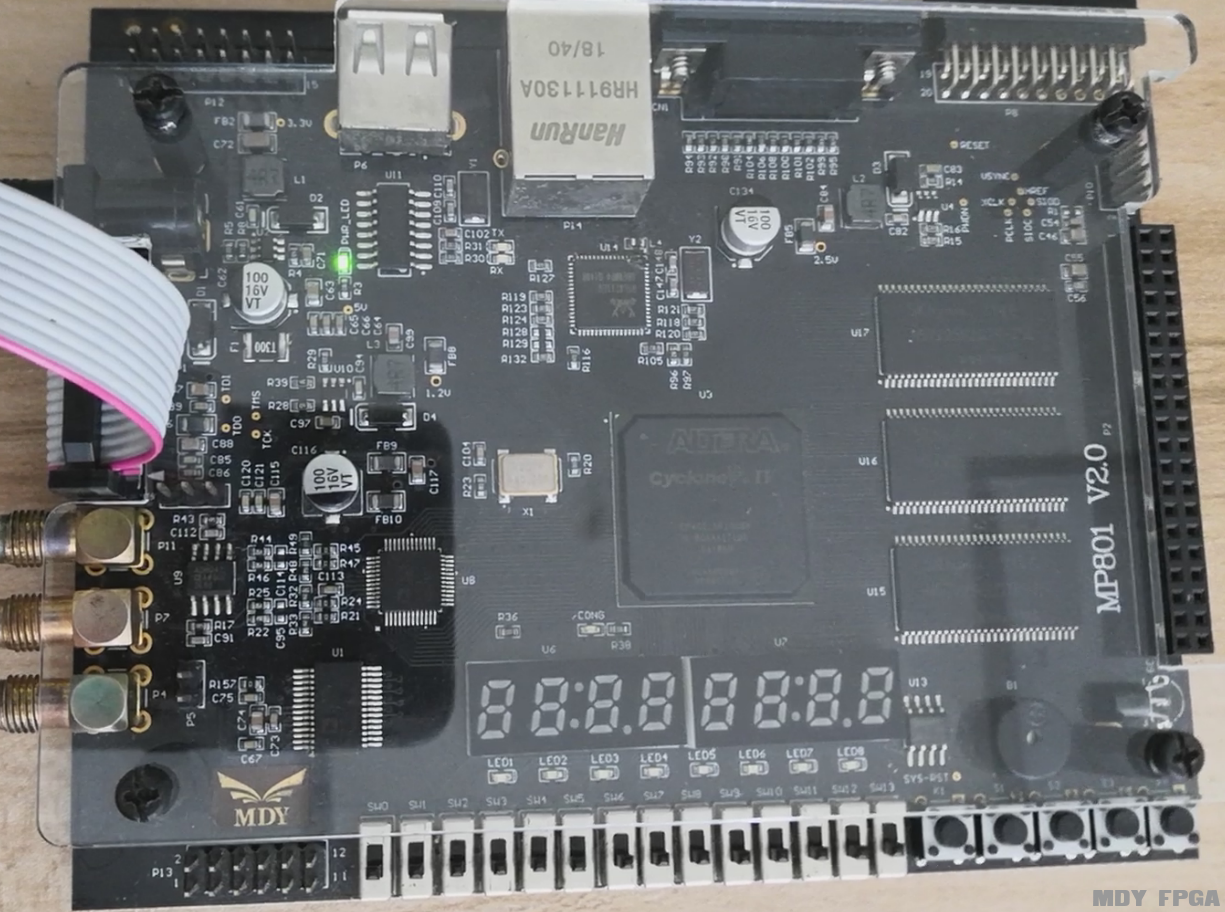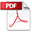# 【每周FPGA案例】PWM流水灯设计

PWM流水灯设计

--作者：小黑同学
【上板现象】

PWM流水灯在MP801的上板现象（点击跳转明德扬论坛观看）

PWM流水灯在点拨开发板的上板现象
（点击跳转明德扬论坛观看）

PWM流水灯在实验箱的上板现象（点击跳转明德扬论坛观看）

【设计教程】

1.1 总体设计

1.1.1 概述

PWM是一种对模拟信号电平进行数字编码的方法。通过使用高分辨率计数器，对方波的占空比进行调制，从而对一个具体模拟信号的电平进行编码。由于在给定的任何时刻，满幅值的直流供电只存在有（ON）和无（OFF）两种状态，因此PWM信号仍然是数字信号。电压或电流源是以一种通（ON）或断（OFF）的重复脉冲序列被加到模拟负载上去的。直流供电被加到负载上的时候为“通”，负载供电被断开的时候为“断”。只要有足够的带宽，任何模拟值都可以使用PWM进行编码。PWM波形图

1.1.2 设计目标

1.1.3信号列表
 信号名 接口方向 定义 clk 输入 系统时钟，50Mhz rst_n 输入 低电平复位信号 led 输出 8位led灯输出信号

1.1.4 设计思路always @(posedge clk or negedgerst_n)begin         if(!rst_n)begin             cnt_1s <= 0;         end         else if(add_cnt_1s)begin             if(end_cnt_1s)                 cnt_1s <= 0;             else                 cnt_1s <= cnt_1s + 1;         end    end       assign add_cnt_1s = 1;    assign end_cnt_1s = add_cnt_1s &&  cnt_1s==50_000_000 -1 ;                always @(posedge clk or negedgerst_n)begin           if(!rst_n)begin             cnt_10s <= 0;         end         else if(add_cnt_10s)begin             if(end_cnt_10s)                 cnt_10s <= 0;             else                 cnt_10s <= cnt_10s + 1;         end    end       assign add_cnt_10s = end_cnt_1s;    assign end_cnt_10s = add_cnt_10s  && cnt_10s==10-1 ;Led~led也是同样，即：

 always   @(posedge clk or negedgerst_n)begin         if(rst_n==1'b0)begin    led <= 0;         end         else if(end_cnt_10s)begin    led <= 0;         end         else if(add_cnt_10s && cnt_10s==1-1)begin    led <= 1;         end    end                always   @(posedge clk or negedgerst_n)begin         if(rst_n==1'b0)begin    led <= 0;         end         else if(end_cnt_10s)begin    led <= 0;         end         else if(add_cnt_10s && cnt_10s==2-1)begin    led <= 1;         end    end                always   @(posedge clk or negedgerst_n)begin         if(rst_n==1'b0)begin    led <= 0;         end         else if(end_cnt_10s)begin    led <= 0;         end         else if(add_cnt_10s && cnt_10s==3-1)begin    led <= 1;         end    end                always   @(posedge clk or negedgerst_n)begin         if(rst_n==1'b0)begin    led <= 0;         end         else if(end_cnt_10s)begin    led <= 0;         end         else if(add_cnt_10s && cnt_10s==4-1)begin    led <= 1;         end    end                always   @(posedge clk or negedgerst_n)begin         if(rst_n==1'b0)begin    led <= 0;         end         else if(end_cnt_10s)begin    led <= 0;         end         else if(add_cnt_10s && cnt_10s==5-1)begin    led <= 1;         end    end                always   @(posedge clk or negedgerst_n)begin         if(rst_n==1'b0)begin    led <= 0;         end         else if(end_cnt_10s)begin    led <= 0;         end         else if(add_cnt_10s && cnt_10s==6-1)begin    led <= 1;         end    end                always   @(posedge clk or negedgerst_n)begin         if(rst_n==1'b0)begin    led <= 0;         end         else if(end_cnt_10s)begin    led <= 0;         end         else if(add_cnt_10s && cnt_10s==7-1)begin    led <= 1;         end    end                always   @(posedge clk or negedgerst_n)begin         if(rst_n==1'b0)begin    led <= 0;         end         else if(end_cnt_10s)begin    led <= 0;         end         else if(add_cnt_10s && cnt_10s==8-1)begin    led <= 1;         end    end

1.1.5参考设计代码

1.2 效果和总结

• 时间经过1秒之后• 时间经过4秒的时候• 时间经过8秒的时候【设计教程下载】PWM流水灯V2.0.pdf (696.4 KB, 下载次数: 0)

【设计视频教程】

【工程源码】01_PWM_waterfall_light.rar (8.23 KB, 下载次数: 0)

# 报名FPGA培训

•
•
•
•
• FPGA教育领域第一品牌
• 咨询热线：020-39002701
• 技术交流Q群：544453837